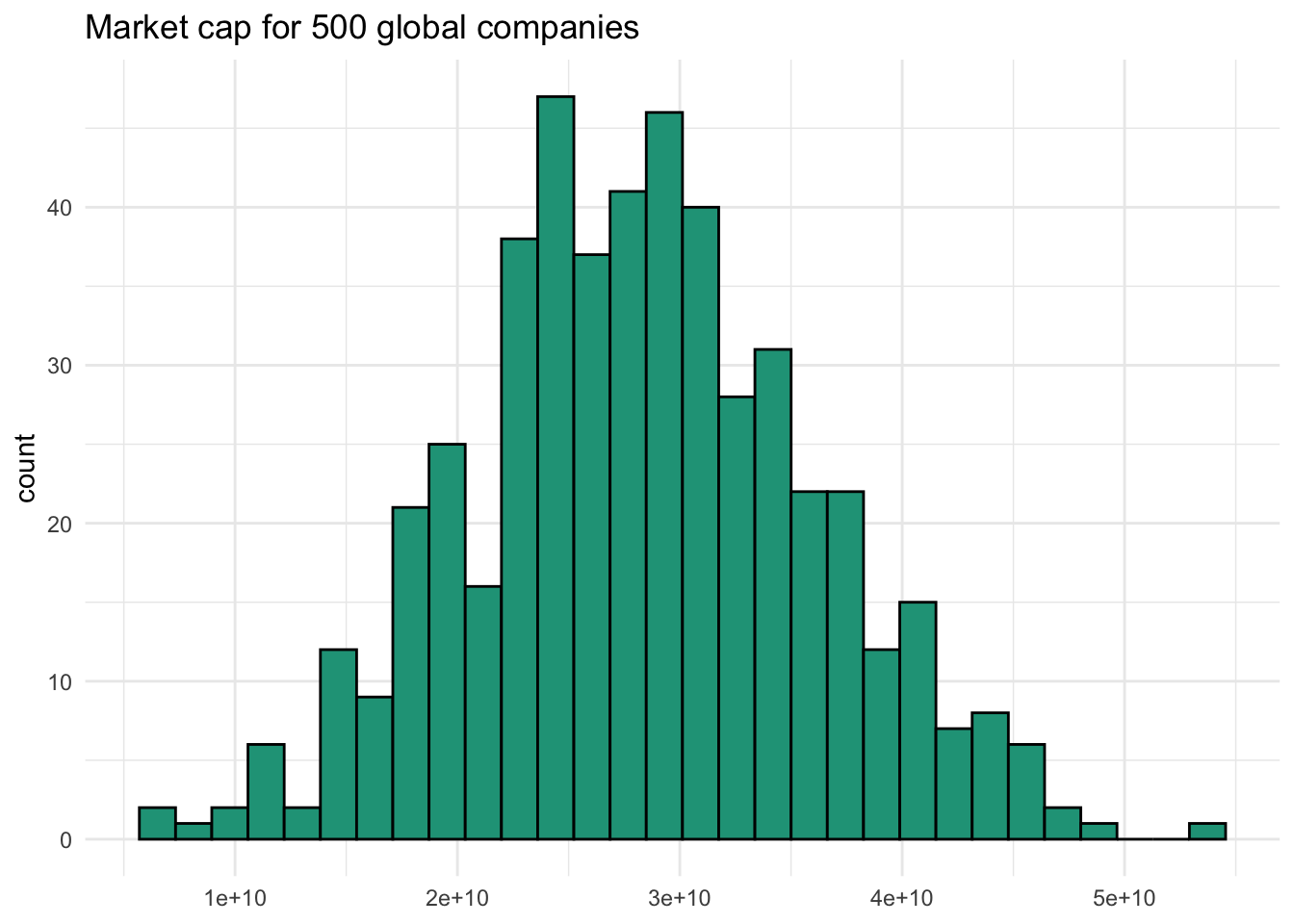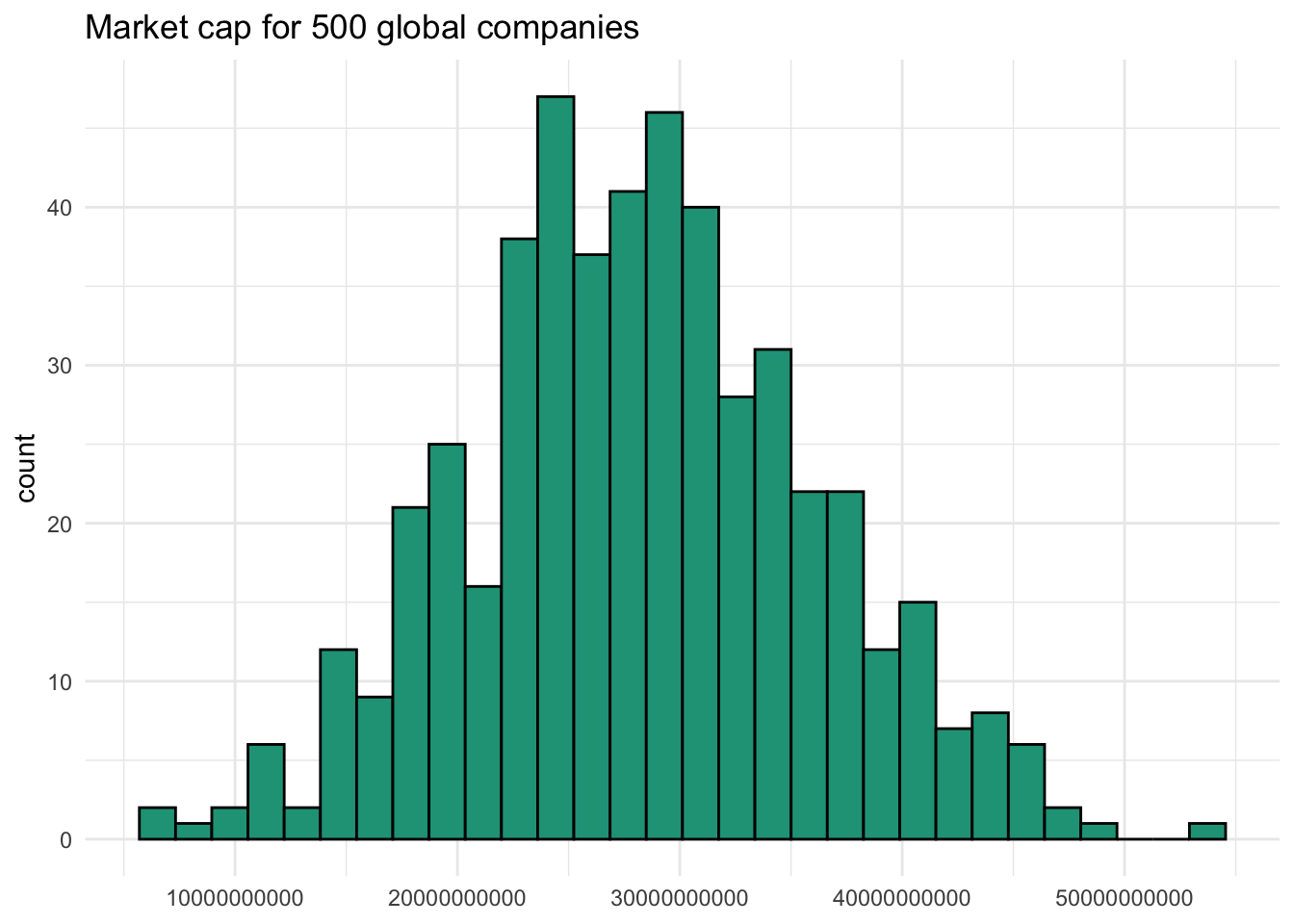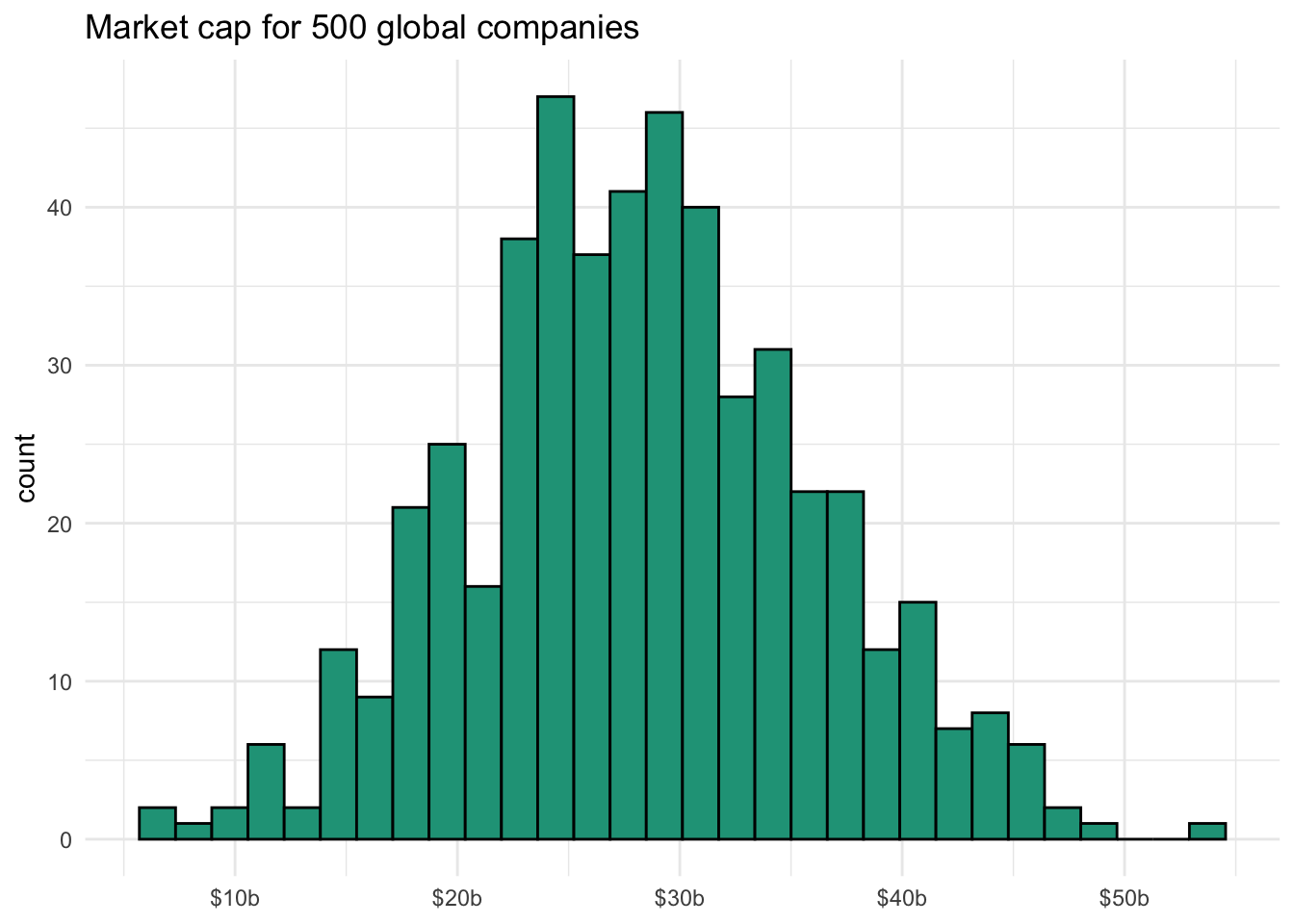# Scientific notation in R

July 11, 2020

Sometimes you work with numbers that are pretty big. The combined GDP of the European Union. The population of India. The number of blog posts debating Python vs. R.

Imagine we’re dealing with 500 global companies that have an average market cap of 28 billion dollars with a standard deviation of 8 billion. Our summary statistics in R would look like this:

``````set.seed(123)
caps <- rnorm(500, 28000000000, 8000000000)
summary(caps)``````
``````##      Min.   1st Qu.    Median      Mean   3rd Qu.      Max.
## 6.713e+09 2.340e+10 2.817e+10 2.828e+10 3.348e+10 5.393e+10``````

It is quite possible that I zoned out during this material while in grade school, but at first glance—and without knowing the dataset well—I find it difficult to interpret the values.

Plotting a histogram with `ggplot2` doesn’t help as it also defaults to scientific notation along the x-axis, although at least it doesn’t pivot from e+09 to e+10 as seen with the summary.

``````library(ggplot2)
ggplot(data.frame(market_cap = caps), aes(x = market_cap)) +
geom_histogram(color = "black", fill = "#1FA187") +
labs(title = "Market cap for 500 global companies",
x =  NULL) +
theme_minimal() ``````## Scientific notation

Before exploring some “hacks” to get rid of the strange notation, let’s take a look at how the translation actually works. Although not always useful, any number can be converted into scientific notation. R uses scientific e notation where e tells you to multiple the base number by 10 raised to the power shown.

Let’s start with the number 28. Scientific notation adds a decimal after the first number before applying the system. So 28 becomes 2.8 x 10^1 or `2.8e+01` in e notation.

If we were interested in a larger number, say 280 million, we’d still use 2.8 and then find the appropriate power to multiply ten by to reach 280,000,000. This would be 2.8 x 10^8 or `2.8e+08`.

The average market cap of \$28b for the 500 companies in our sample would require 2.8 x 10^10. So `2.8e+10` is equivalent to 28,000,000,000.

Here are the variants with a base 2.8 up through 10^10.

``````library(knitr)
sci <- data.frame(sci_note = 2.8 * 10^(1:10))
sci <- sci %>% mutate(translation = format(sci_note, scientific = FALSE, big.mark = ","))
kable(sci, col.names = c("Scientific Notation", "Full digit equivalent"))``````

Scientific NotationFull digit equivalent
2.8e+0128
2.8e+02280
2.8e+032,800
2.8e+0428,000
2.8e+05280,000
2.8e+062,800,000
2.8e+0728,000,000
2.8e+08280,000,000
2.8e+092,800,000,000
2.8e+1028,000,000,000

## Side-stepping the interpretation problem

### Targeted

If you want to avoid scientific notation for a given number or a series of numbers, you can use the `format()` function by passing `scientific = FALSE` as an argument.

``````big_number <- 28000000000
big_number``````
``##  2.8e+10``
``format(big_number, scientific = FALSE)``
``##  "28000000000"``

You can address the newly surfaced visual problem of too many zeros by adding comma separators with `big.mark = ','`

``format(big_number, scientific = FALSE, big.mark = ',')``
``##  "28,000,000,000"``

### Universal

I generally apply the more aggressive solution by telling R to avoid all scientific notation by setting `options(scipen=999)` at the top of the script. This forces the full display.

``````options(scipen=999)
format(summary(caps), big.mark = ",") # using big.mark to add commas``````
``````##             Min.          1st Qu.           Median             Mean          3rd Qu.
## " 6,712,617,612" "23,402,938,762" "28,165,737,180" "28,276,723,580" "33,481,690,122"
##             Max.
## "53,928,319,480"``````

After adding this bit of code, our histogram includes all the expected digits by default.

``````ggplot(data.frame(market_cap = caps), aes(x = market_cap)) +
geom_histogram(color = "black", fill = "#1FA187") +
labs(title = "Market cap for 500 global companies",
x =  NULL) +
theme_minimal() ``````Is this easier to read? Probably not, even if commas were added to breakup all those zeros. Thankfully we can use `library(scales)` to convert the axis labels to something more visually appealing. Here we call `scale_x_continuous` and pass the `label_number` function along with `scale = 1e-9` in the `labels` argument. This tells the computer to scale back the data by 10^-9.

``````library(scales)

ggplot(data.frame(market_cap = caps), aes(x = market_cap)) +
geom_histogram(color = "black", fill = "#1FA187") +
labs(title = "Market cap for 500 global companies",
x =  NULL) +
theme_minimal() +
scale_x_continuous(labels  =
label_number(scale = 1e-9, prefix = "\$", suffix = "b", accuracy = 1)) ``````We can see this scaling work with our `big_number` variable of 28 billion, reducing it to the expected value of 28.

``big_number * 10^-9``
``##  28``

## Reverting back

You can toggle between scientific notation preferences in chunks of code by turning it off as described above with `options(scipen=999)` and reactivating it with `options(scipen=0)`.

``````options(scipen=0)
big_number``````
``##  2.8e+10``Or create a free DataKwery.com account

### Related Courses

edX
Harvard University
Free
Data Science: R Basics
Rafael A. Irizarry8 weeks, 2 hours/weekBeginner

### Related Learning Paths

Coursera
Johns Hopkins University

5 Courses 7 Months